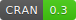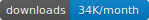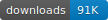# D3 JavaScript Network Graphs from R

Version 0.3This README includes information on set up and a number of basic examples. For more information see the package's main page.

## Usage

Here's an example of `simpleNetwork`:

``````library(networkD3)

# Create fake data
src <- c("A", "A", "A", "A", "B", "B", "C", "C", "D")
target <- c("B", "C", "D", "J", "E", "F", "G", "H", "I")
networkData <- data.frame(src, target)

# Plot
simpleNetwork(networkData)``````

Here's `forceNetwork`:

``````# Load data
data(MisNodes)

# Plot
forceNetwork(Links = MisLinks, Nodes = MisNodes, Source = "source",
Target = "target", Value = "value", NodeID = "name",
Group = "group", opacity = 0.4,
colourScale = JS("d3.scaleOrdinal(d3.schemeCategory20);"))``````

Here's `sankeyNetwork` using a downloaded JSON data file:

``````# Recreate Bostock Sankey diagram: http://bost.ocks.org/mike/sankey/
# Load energy projection data
URL <- paste0("https://cdn.rawgit.com/christophergandrud/networkD3/",
"master/JSONdata/energy.json")
Energy <- jsonlite::fromJSON(URL)

# Plot
sankeyNetwork(Links = Energy\$links, Nodes = Energy\$nodes, Source = "source",
Target = "target", Value = "value", NodeID = "name",
units = "TWh", fontSize = 12, nodeWidth = 30)``````

### Interacting with igraph

You can use igraph to create network graph data that can be plotted with networkD3. The `igraph_to_networkD3` function converts igraph graphs to lists that work well with networkD3. For example:

``````# Load igraph
library(igraph)

# Use igraph to make the graph and find membership
karate <- make_graph("Zachary")
wc <- cluster_walktrap(karate)
members <- membership(wc)

# Convert to object suitable for networkD3
karate_d3 <- igraph_to_networkD3(karate, group = members)

# Create force directed network plot
Source = 'source', Target = 'target', NodeID = 'name',
Group = 'group')``````

### Saving to an external file

Use `saveNetwork` to save a network to stand alone HTML file:

``````library(magrittr)

simpleNetwork(networkData) %>% saveNetwork(file = 'Net1.html')``````

## Note

networkD3 began as a port of d3Network package to the htmlwidgets framework. d3Network is no longer supported.